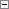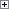A FlowField is always associated with a calculation formula that determines how the FlowField is calculated. The following syntax is valid for the CalcFormula property.Copy Code
<CalculationFormula> ::=
[-]Exist(<TableNo> [WHERE (<TableFilters>)]) |
Count(<TableNo> [WHERE (<TableFilters>)]) |
[-]Sum(<TableNo>.<FieldNo> [WHERE(<TableFilters>)])|
[-]Average(<TableNo>.<FieldNo> [WHERE (<TableFilters>)]) |
Min(<TableNo>.<FieldNo> [WHERE (<TableFilters>)]) |
Max(<TableNo>.<FieldNo> [WHERE (<TableFilters>)]) |
Lookup(<TableNo>.<FieldNo> [WHERE (<TableFilters>)])
<TableFilters> ::=
[<TableFilter> {,<TableFilter>}]
<TableFilter> ::=
<DstFieldNo>=CONST(<FieldConst>) |
<DstFieldNo>=FILTER(<Filter>) |
<DstFieldNo>=FIELD(<SrcFieldNo>) |
<DstFieldNo>=FIELD(UPPERLIMIT(<SrcFieldNo>)) |
<DstFieldNo>=FIELD(FILTER(<SrcFieldNo>)) |
<DstFieldNo>=FIELD(UPPERLIMIT(FILTER(<SrcFieldNo>)))

Parts of the formula are described in the following table.

Symbol Description

<TableNo>

Specifies the table holding the information to be used in the FlowField.

<FieldNo>

Specifies the column from which you want to compute values.

<TableFilters>

A list of filters to be used in the computation of the FlowField.

<TableFilter>

A table filter can be one of the following: a constant expression, a filter expression, a value from ordinary fields, or a FlowFilter field. A key for the other table must exist and include the fields used in the filters.

<DstFieldNo>

Specifies the destination field number.

<SrcFieldNo>

Specifies the source field number.

<Filter>

A filter expression such as 10|20..30.